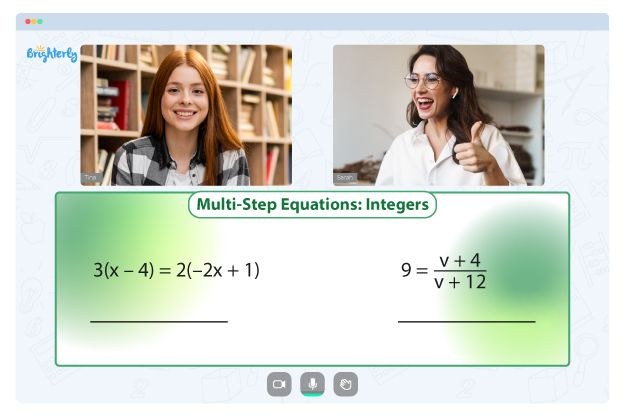# Rule of Exponents Worksheets

Almost all basic operations in math can be simplified using exponents, which significantly reduces the steps you will need to perform for these operations. For example, you can use exponents to decomplexify processes with fractions, roots, and decimals.

Here is everything you need to know about the rules and law of exponents worksheet and how the rules of exponent worksheets can help you.

## What are the rules of exponents?

Rules of exponents are also called laws of exponents or properties of exponents, depending on who uses them. Simplifying expressions using exponents becomes more complex in problems like fractions, roots, irrational numbers, and decimals. You need to know the rules when working with fractions, decimals, irrational numbers, and negative integers as their exponents.

Here are some rules of exponents and how they work with operations.

For example, a rule of exponents states that am × an = am + n. When encountering a problem that says 53 × 52, you have to use the exponent rules and you will get 53 × 52 =  53+2 =  55.

This rule is not restricted to smaller numbers; you can also solve for larger ones using the same rules.

Here is a list of the rules/laws/properties of exponents. Some of them have names/tags that they go by for easy recognition and understanding.

• Zero exponent rule: a0 = 1
• Identity exponent rule: a1 = a
• Product rule: am × an = am+n
• Quotient rule: am / an = am−n
• Negative exponent rule: a−m = 1/am
• Power of a power rule: (am)n = amn
• Power of a product rule: (ab)m = ambm
• Power of a quotient rule: (a/b)m = am/bm
• Fractional exponents rule:  a1/n = n√a

When you look at all these rules, you see how easy it can be for a child to get confused as each of these rules has its meaning and represents the exponent universe. Therefore, kids will find the rules of exponents worksheets very beneficial.

## Benefits of exponent rules worksheet

Here are some of the benefits of exponent rules worksheets:

Math for Kids

Is Your Child Struggling With Math?
1:1 Online Math Tutoring### Kids remember the rules

The easiest way to understand and tackle a math problem is by understanding and remembering the rules attached to that problem. With the laws of exponents worksheet, kids get to practice the rules of exponents now and then, and they get familiar with the properties.

They must remember the laws when faced with an exponent-based problem in a speed test or exam. You can also develop a study practice system with your kids using the exponent rules review worksheet and the exponent rules review worksheet answers.

### The process of solving exponents is simplified

The exponent laws worksheet breaks down the process into recognizable formats for your kid.### Law of Exponents Worksheet PDF

Law of Exponents Worksheet### Exponent Rules Worksheet PDF

Exponent Rules Worksheet### Exponent Rules Review Worksheet PDF

Exponent Rules Review Worksheet### Exponent Laws Worksheet PDF

Exponent Laws Worksheet

Problems with Equations?• Start learning with an online tutor.

Is your child finding it challenging to grasp of equations? An online tutor could be the answer.